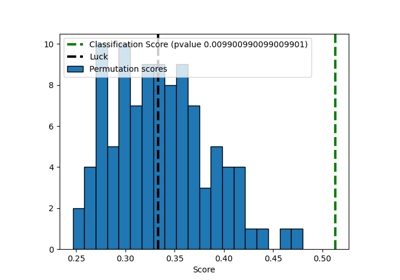# sklearn.model_selection.permutation_test_score¶

sklearn.model_selection.permutation_test_score(estimator, X, y, *, groups=None, cv=None, n_permutations=100, n_jobs=None, random_state=0, verbose=0, scoring=None)[source]

Evaluate the significance of a cross-validated score with permutations

Read more in the User Guide.

Parameters
estimatorestimator object implementing ‘fit’

The object to use to fit the data.

Xarray-like of shape at least 2D

The data to fit.

yarray-like of shape (n_samples,) or (n_samples, n_outputs) or None

The target variable to try to predict in the case of supervised learning.

groupsarray-like of shape (n_samples,), default=None

Labels to constrain permutation within groups, i.e. y values are permuted among samples with the same group identifier. When not specified, y values are permuted among all samples.

When a grouped cross-validator is used, the group labels are also passed on to the split method of the cross-validator. The cross-validator uses them for grouping the samples while splitting the dataset into train/test set.

scoringstr or callable, default=None

A single str (see The scoring parameter: defining model evaluation rules) or a callable (see Defining your scoring strategy from metric functions) to evaluate the predictions on the test set.

If None the estimator’s score method is used.

cvint, cross-validation generator or an iterable, default=None

Determines the cross-validation splitting strategy. Possible inputs for cv are:

• None, to use the default 5-fold cross validation,

• int, to specify the number of folds in a (Stratified)KFold,

• An iterable yielding (train, test) splits as arrays of indices.

For int/None inputs, if the estimator is a classifier and y is either binary or multiclass, StratifiedKFold is used. In all other cases, KFold is used.

Refer User Guide for the various cross-validation strategies that can be used here.

Changed in version 0.22: cv default value if None changed from 3-fold to 5-fold.

n_permutationsint, default=100

Number of times to permute y.

n_jobsint, default=None

The number of CPUs to use to do the computation. None means 1 unless in a joblib.parallel_backend context. -1 means using all processors. See Glossary for more details.

random_stateint, RandomState instance or None, default=0

Pass an int for reproducible output for permutation of y values among samples. See Glossary.

verboseint, default=0

The verbosity level.

Returns
scorefloat

The true score without permuting targets.

permutation_scoresarray of shape (n_permutations,)

The scores obtained for each permutations.

pvaluefloat

The p-value, which approximates the probability that the score would be obtained by chance. This is calculated as:

(C + 1) / (n_permutations + 1)

Where C is the number of permutations whose score >= the true score.

The best possible p-value is 1/(n_permutations + 1), the worst is 1.0.

Notes

This function implements Test 1 in:

Ojala and Garriga. Permutation Tests for Studying Classifier Performance. The Journal of Machine Learning Research (2010) vol. 11 [pdf].

## Examples using sklearn.model_selection.permutation_test_score¶